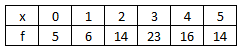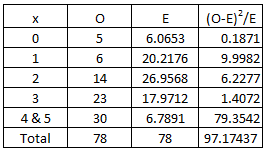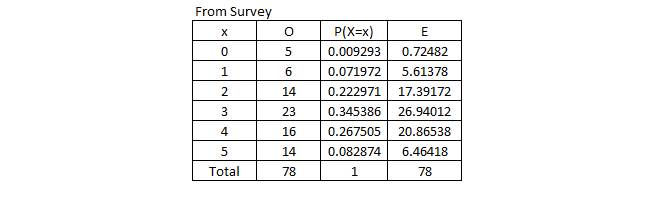Select Page

STPM 2018 term 3 mathematics (T) Coursework sample

Guideline for solving STPM 2018 Term 3 Mathematics (T) PBS coursework assignment

methodology

tabulate

Thanks for helping in doing the survey.

You can find the sample of the results below. Scroll down for all the sample answers and solutions.

Question

A researcher claims that 40% of Malaysians wear glasses. The number of people wearing glasses in a family may be modelled by a binomial distribution. In this assignment, you are required to conduct a study on the number of family members wearing glasses in k-member families with k>4.

1 Comment on the suitability of the binomial model in this situation.

2 By choosing one value of k, take a random sample of at least 50 families with k members. Tabulate the distribution of the number of family members wearing glasses.

3 Test the hypothesis that your sample data is from a binomial distribution with parameter p = 0.4.

4 (a) Explain how the test would be modified if the hypothesis to be tested that your sample data is from a binomial distribution with the parameter p unknown.
(b) Carry out this test.

5 Comment on your results.

I have conducted survey on the number of family members wearing glasses in 5-member families. The method i used to conduct the survey is using the Google Form. Thanks to the students who helped to fill in the form.

1

i. Each family member (trial) has two outcomes; wearing glasses and not wearing glasses.
ii. Fixed number of family members. (5 members)
iii. Independent, The probability of family member wearing glasses is assumed to be independent. We neglect the factors like genetic, races and sex
iv. The probability of success, p, is assumed to be constant for each family member.

2A total of 78 5-members families are chosen. The frequency distributions are tabulated as above.

3

Chi-squared test is used to test if the sample data is from binomial distribution with parameter p=0.4.

Significance level chosen is 5%.

H0: The number of family members who are wearing spectacles can be modelled by a binomial distribution with parameter p=0.4.

H1: The number of family members who are wearing spectacles cannot be modelled by a binomial distribution with parameter p=0.4.The values of P(X=x) is obtained using the formula of binomial distribution.

The values of E can be obtained by taking the P(X=x) and multiply with 78 (Total families in survey)

The survey was conducted using Google Form and all students are allowed to fill in the form with multiple times (even though they have been warned to fill in once). Therefore the data i used above is taken exactly from the survey from students.

The expected frequency of the category x=5 is less than 5. Therefore the categories x=4 and x=5 are combined.

Please take note that the expected frequencies should not be less than 5.Degree of freedom, v=n-1.

(n=the number of categories, 1=the number of restrictions)

For my case, v=5-1=4.

You can refer to the chi-squared distribution table. For my case, we test using 5% significance level, (we read 0.95 from the table), critical value = 9.488.

We reject H0 if the test statistic is larger than 9.488.

Test statistic = 97.17 (based on the table above).

Since the test statistic 97.17 > 9.488. H0 is rejected and it is enough evidence at 5% significance level, the data does not fit binomial distribution with parameter p=0.4.

Even at 0.1% significance level, H0 is also rejected because the test statistic is large.

4

(a) When the parameter, p is unknown, we have to estimate the p by using the property of mean of binomial = mean of data.

Another modification will be the degree of freedom. The number of restrictions is 2 now. So degree of freedom = n-2.

(b) We have to find mean from the data obtained from the survey. Please refer the table below.

Mean = 237/78=3.0384615384615384615384615384615

Mean of binomial = np = 5p

5p =  3.0384615384615384615384615384615

p=0.6076923H0: The number of family members who are wearing spectacles can be modelled by a binomial distribution with parameter p=0.6076923.

H1: The number of family members who are wearing spectacles cannot be modelled by a binomial distribution with parameter p=0.6076923.

Significance level chosen is 5%.The values of P(X=x) is obtained using the formula of binomial distribution, X ~ B (5, 0.6076923).

The values of E can be obtained by taking the P(X=x) and multiply with 78 (Total families in survey)

The survey data is the same as the Question 3.

The expected frequency of the category x=0 is less than 5. Therefore the categories x=0 and x=1 are combined.

Please take note that the expected frequencies should not be less than 5.Degree of freedom, v=n-2.

(n=the number of categories, 1=the number of restrictions)

For my case, v=5-2=3.

You can refer to the chi-squared distribution table. For my case, we test using 5% significance level, (we read 0.95 from the table), critical value = 7.815.

We reject H0 if the test statistic is less than 7.815.

Test statistic = 14.58534 (based on the table above).

Since the test statistic 14.58534 > 7.815. H0 is rejected and it is enough evidence at 5% significance level, the data does not fit binomial distribution with parameter p=0.6076923.

But at 0.1% significance level, H0 is not rejected because the test statistic is smaller compare the critical value (16.27).

5

At any level of significance, the sample data does not fit binomial distribution with p = 0.4.
The Chi-square test show that the sample data does not fit binomial distribution with p = 0.6076923 for any level of significance, except 0.1%.Subscribe To KK LEE Newsletter

Join KKL EE mailing list to receive the latest news and updates from the team. Get notified when new articles (including pbs sample) are posted.